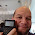## Saturday, April 3, 2021

### Carnival of Mathematics #192

Carnival of Mathematics #192

Welcome to a very special edition of Eddie's Math and Calculator Blog.  Today I am hosting the 192nd Carnival of Mathematics, which is hosted and facilitated by The Aperiodical.  You can check out all of the carnivals, plus submit your entries to be featured in the monthly series at https://aperiodical.com/carnival-of-mathematics/.  Special thanks to Katie Steckles, Christian Perfect, and Peter Rowlett.

The Carnival of Mathematics is a monthly blog.   Please check out March's Carnival (191) by Fractal Kitty.  May's Carnival (193) will be hosted by Ben from Math off the grid.

The Number 192

Per tradition, every Carnival looks at the number associated with the month's entry, and mine is 192.  192 is an even integer and the factorization is 2^6 × 3.  The divisors of 192 are: 1, 2, 3, 4, 6, 8, 12, 16, 24, 32, 48, 64, 96, and 192.  Adding up the proper divisors (all the divisors except the number itself) will get us the sum of 316, which makes 192 an abundant number.

On the calendar, the 192nd day of the year is July 11.  On leap years, the 192nd days of the year becomes July 10.  192 hours converts to 8 days.

192 can be expressed as sums and differences of squares, such as:

192 = 1^2 + 3^2 + 5^2 + 6^2 + 11^2
192 = 1^2 + 5^2 + 6^2 + 7^2 + 9^2
192 = 14^2 - 2^2
192 = 15^2 - (7^2 - 4^2)

The Carnival

Our first entry comes from Gödel's Lost Letter and P=NP.   This blog entry is all about the Rubik's Cube, featuring the decades-long interest by the Wall Street Journal, and the Distinguished Professor and speed-Rubik's Cube champion Jessica Fridrich.  The blog entry gives an introductory look on how the Rubik's Cube lends to group theory.

In his entry, Stacking LEGO BricksPeter Kagey uses combinatorics, with a simple set of rules, to demonstrate how many ways 2 x n LEGO pieces can be stacked, and how it is related to the function 3^(n-1).

Next up is a great video by Ganit Charcha titled A Walk Through Number Land. Highlights include the multiplicative properties of 1089 and 142857, Harshad numbers, Taxicab numbers, Kaprekar numbers, Happy numbers, and palindromic numbers.

Ganit Charcha submitted a second video, Colour Coding of some Decimal Representations - An Interesting Activity, where each digit of π, 1/3, 1/7, and other non-integers is giving its own color.  I like how color coding gives us a visual representation of numbers.

Next up is a four part series by Lisa Charlotte Rost:

The four part blog discusses various strategies on how to use color scales to illustrate statistical data.  The series has illustrations to demonstrate which scales work the best (and not so much) for each situation.  I recommend this series to business and finance students and professionals.

Anna of One + εpsilon created a mathematical board game based on the 3N + 1 Conjecture.  The 3N + 1 conjecture is consists of one of two steps based on whether the integer N is even or odd:  if N is even, divide it by 2.  If N is odd, multiply by 3 and add 1.  The goal is to reduce N to one.  The board game is presented as a PDF file and it is a great way to engage mathematics students.

Thank you to everyone who submitted articles, and to Aperiodical for inviting me to host a Carnival!

Until next time,

Eddie

All original content copyright, © 2011-2021.  Edward Shore.   Unauthorized use and/or unauthorized distribution for commercial purposes without express and written permission from the author is strictly prohibited.  This blog entry may be distributed for noncommercial purposes, provided that full credit is given to the author.

1.Thanks for all the great links. I'll be using them in some of the courses I'm taking.

2.For the second video that we had submitted please mention Ganit Charcha. It has been written as "Charcha submitted a second video... "

1.It should read Ganit Charcha now. Thank you,

Eddie

3.A nice relationship about 192 that students might be given, with a power of two relationship they might figure out: Because 192 is divisible by four, it is the difference of two squares n^2 and (n+2)^2, 49^2 - 47^2; because it is divisible by 8, it is the difference of two squares that n^2 + (n+4)^2; 26^2 - 22^2. But don't stop there, because it is divisible by 16, it is the difference of two squares n^2 and (n+6)^2; 19^2 - 13^2 , and YES, it it also divisible by 32, so one more time, 16^2-8^2.

### Σ(1 / (a^n)) from n=1 to m

Σ(1 / (a^n)) from n=1 to m This blog entry covers the sum of the series: Σ[1 / (a^n), n=1 to m] with n and m positive integers Specific Cas...